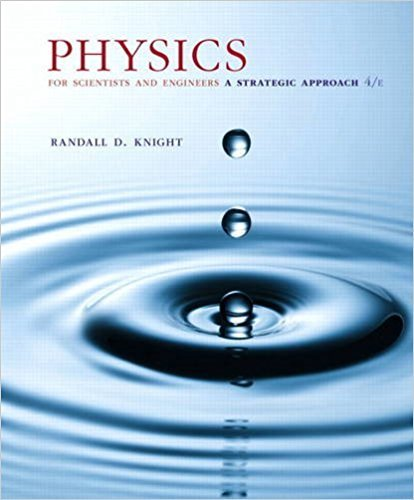×

# Solved: The atomic mass number of copper is A = 64. AssumeISBN: 9780134081496 191

## Solution for problem 18.78 Chapter 18

Physics for Scientists and Engineers: A Strategic Approach, Standard Edition (Chs 1-36) | 4th Edition

• Textbook Solutions
• 2901 Step-by-step solutions solved by professors and subject experts
• Get 24/7 help from StudySoup virtual teaching assistantsPhysics for Scientists and Engineers: A Strategic Approach, Standard Edition (Chs 1-36) | 4th Edition

4 5 0 270 Reviews
21
5
Problem 18.78

The atomic mass number of copper is A = 64. Assume that atoms in solid copper form a cubic crystal lattice. To envision this, imagine that you place atoms at the centers of tiny sugar cubes, then stack the little sugar cubes to form a big cube. If you dissolve the sugar, the atoms left behind are in a cubic crystal lattice. What is the smallest distance between two copper atoms?

Step-by-Step Solution:
Step 1 of 3

Physics 105 Week 3 Notes By Lauren Faris Chapter 2: 1­D Kinematics (Continued)  The position of a particle can be described as a position vector.  Average velocity is the displacement divided by the time interval.  Instantaneous velocity is the instantaneous rate of change of position vector with respect to time. It is always tangent to its path.  Acceleration describes...

Step 2 of 3

Step 3 of 3

##### ISBN: 9780134081496

Unlock Textbook Solution

Solved: The atomic mass number of copper is A = 64. Assume

×